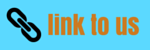# Reflection of light Notes for Class 10

## Spherical Mirrors

• The reflecting surface of a spherical mirror may be curved inwards or outwards.
• Spherical mirrors are of two types
1. Concave mirror: - In a concave mirror reflection of light takes place at the concave surface or bent-in surface as shown below in the figure.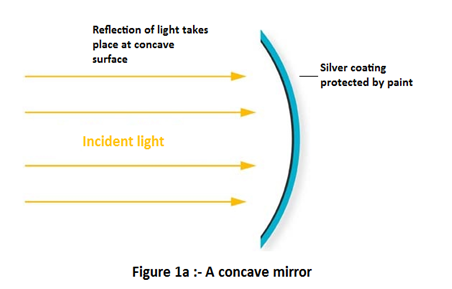2. Convex mirror:- In a convex mirror reflection of light takes place at the convex surface or bent out surface as shown below in the figure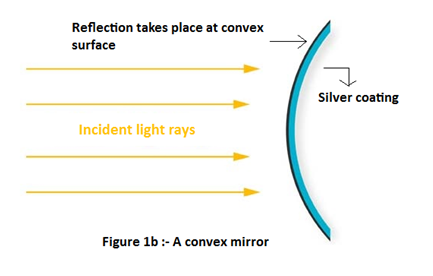• Commonly used terms about Spherical mirrors :-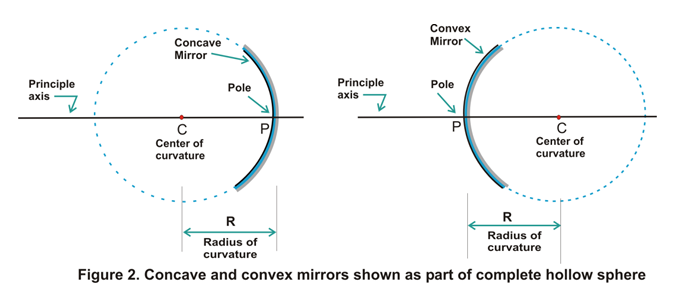1. Centre of curvature: - The reflecting surface of a spherical mirror forms a part of a sphere. This sphere has a centre. This point is called the centre of curvature of the spherical mirror. It is represented by the letter C. Please note that the centre of curvature is not a part of the mirror. It lies outside its reflecting surface. The centre of curvature of a concave mirror lies in front of it. However, it lies behind the mirror in case of a convex mirror as shown above in the figure 2.
2. Radius of curvature: - The radius of the sphere of which the reflecting surface of a spherical mirror forms a part, is called the radius of curvature of the mirror. It is represented by the letter R.
3. Pole: - The center of a spherical mirror is called its pole and is represented by letter P as can be seen in figure 2.
4. Principle axis: - Straight line passing through the pole and the centre of curvature of a spherical mirror is called principle axis of the mirror.
5. Aperture of the mirror: - Portion of the mirror from which reflection of light actually takes place is called the aperture of the mirror. Aperture of the mirror actually represents the size of the mirror.

## Principle focus and focal length of a Spherical Mirrors

• For understanding about principle focus and focus length of a spherical mirror first consider the figure given below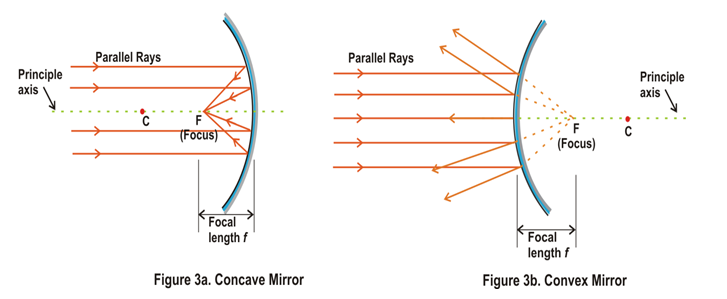• From figure 3a we see that a number of rays parallel to the principal axis are falling on a concave mirror. If we now observe the reflected rays we see that they are all intersecting at a point F on the principal axis of the mirror. This point is called the principal focus of the concave mirror.
• In case of convex mirror rays get reflected at the reflecting surface of the mirror and these reflected rays appear to come from point F on the principle axis and this point F is called principle focus of convex mirror.
• The distance between the pole and the principal focus of a spherical mirror is called the focal length. It is represented by the letter f.
• There is a relationship between the radius of curvature R, and focal length f, of a spherical mirror and is given by R=2f which means that that the principal focus of a spherical mirror lies midway between the pole and centre of curvature.